## 4.4Wall boundaries

The no-slip condition is generally applied at a solid wall which is impermeable (assuming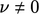). The condition is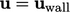, where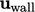is the velocity of the wall, which is usually stationary with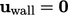. The proof behind the no-slip condition is that it predicts a pressure drop along tubes of small diameter which matches experiments.3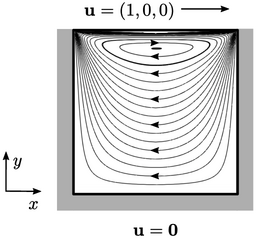The 2D, lid-driven cavity is a ﬂow problem in which no-slip conditions are applied at all boundaries. It provides insight into the boundary condition for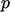at a wall. From Eq. (2.47 ) for an incompressible ﬂuid, with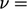constant, the component normal to the domain boundary is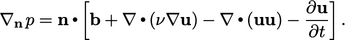(4.4)
At the solid wall boundary, the last 2 terms in Eq. (4.4) disappear since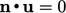, reducing the gradient condition to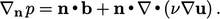(4.5)
The second term can be written (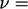constant)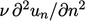, so is only non-zero where there is ﬂow normal to the boundary in its vicinity, e.g. at the corners of the cavity in our example. The term is usually small and its calculation involves extrapolation from the internal solution which often causes instability, so it is generally ignored.

A body force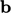, e.g. gravity, is generally prescribed so it does not introduce instability. Where it is signiﬁcant, it must be included in the boundary condition, i.e.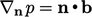.

Otherwise, in the absence of a body force, we reach the standard form of boundary condition for pressure at a wall,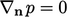.

### Fixing pressure

With only ﬁxed gradient conditions on pressure at the boundary, the pressure value is not ﬁxed at any point in solution domain. The solution is not unique, as shown in the 1D example below with gradient conditions at both ends.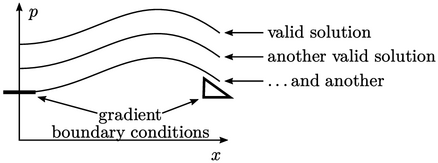To achieve a unique solution,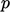must then be ﬁxed to a reference value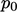at a reference cell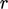in the domain. To achieve this, the diagonal coeﬃcient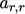is doubled and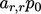is added to source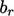, in the matrix equation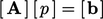described by Eq. (3.1 ). This minimal change “pins” the solution to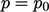in cell.

3Jean Poiseuille, Recherches expérimentales sur le mouvement des liquides dans les tubes de très petits diamètres I-IV, 1840.

Notes on CFD: General Principles - 4.4 Wall boundaries